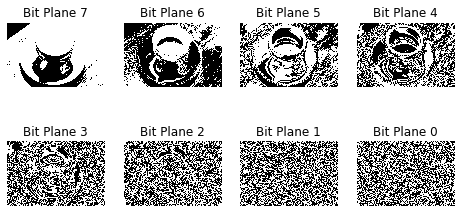## 数字图像位平面分解

$$f_{k}^{n}{(x,y)} = f_{k}(x,y) {\&} 2^{n} \quad (n = 0, \ldots, 7)$$

## 用 python 来实现数字图像位平面分解

pip install matplotlib numpy scikit-image


import matplotlib.pyplot as plt
import numpy as np
from skimage import data


def bitplane(img:np.ndarray):
shape = img.shape
bits = np.unpackbits(img.reshape(-1, 1), axis=1)
plt.figure(figsize=(8,4))
for i in range(8):
bit_plane_i = bits[:,i].reshape(shape)
plt.subplot(2,4,i+1)
plt.imshow(bit_plane_i, cmap="gray")
plt.title('Bit Plane {}'.format(7-i))
plt.axis('off')


image = data.coffee()

plt.imshow(image);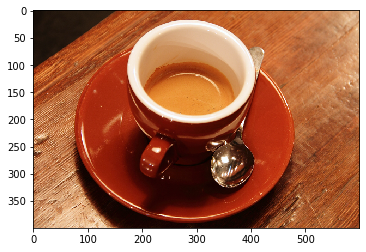B = image[:,:,0]
G = image[:,:,1]
R = image[:,:,2]

plt.figure(figsize=(10,8))
plt.subplot(1,3,1)
plt.imshow(R, cmap="gray")
plt.title('R')

plt.subplot(1,3,2)
plt.imshow(G, cmap="gray")
plt.title('G')

plt.subplot(1,3,3)
plt.imshow(B, cmap="gray")
plt.title('B');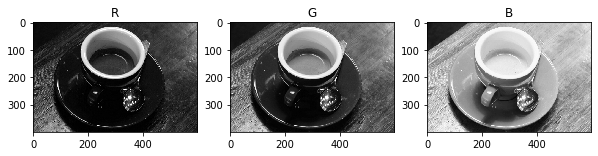bitplane(R)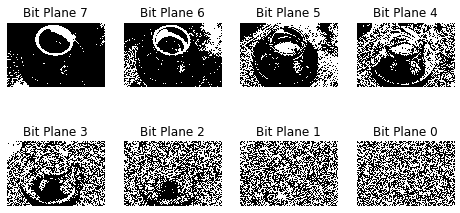bitplane(G)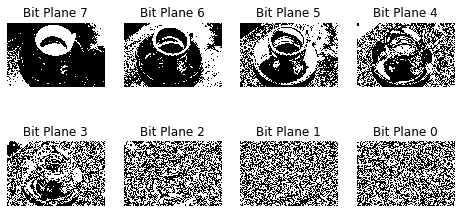bitplane(B)